Irreducible implies primary (Noetherian)

Statement

In a Noetherian ring, any irreducible ideal is primary.

Proof

Hands-on proof

Let$R$ be a Noetherian ring and$P$ an irreducible ideal in$R$. Suppose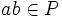$ab \in P$ but$a \notin P$. We need to show that there exists a$n$ such that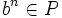$b^n \in P$.

Define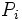$P_i$ as the ideal of all elements$x$ such that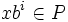$xb^i \in P$. Clearly we get an ascending chain of ideals in$R$: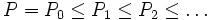$P = P_0 \le P_1 \le P_2 \le \ldots$

Since$R$ is Noetherian, there exists a natural number$n$ such that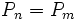$P_n = P_m$ for all$m \ge n$. In other words, there exists a$n$ such that if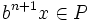$b^{n+1}x \in P$, then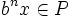$b^nx \in P$ for any$x \in R$.

Now let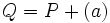$Q = P + (a)$ and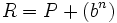$R = P + (b^n)$. Clearly,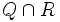$Q \cap R$ contains$P$. We want to show that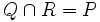$Q \cap R = P$.

For this, suppose$y \in Q \cap R$. Then we can write: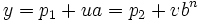$y = p_1 + ua = p_2 + vb^n$

where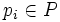$p_i \in P$. This tells us that: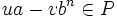$ua - vb^n \in P$.

multiplying both sides by$b$ we get:$uab - vb^{n+1} \in P$

By our assumption,$ab \in P$ and hence$uab \in P$.

This gives us: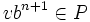$vb^{n+1} \in P$

but from the property of$n$,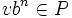$vb^n \in P$ and hence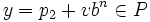$y = p_2 + vb^n \in P$.

Thus any element of$Q \cap R$ is in$P$, so$Q \cap R = P$.

Comparison with the proof for principal ideal rings

In the case of principal ideal rings, we employ a very similar proof, except that the$n$ is replaced by 1.